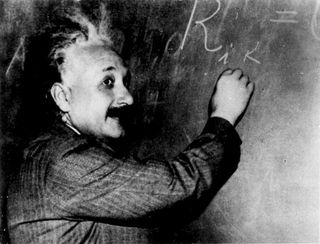# Math Idol: Voters Pick Greatest EquationsIf the writers of equations had an Academy Awards or a Pullitzer Prize, the finalists might be Maxwell, Euler, Newton, Einstein, and whoever can take credit for '1+1=2.'

Robert Crease, a professor of philosophy at the State University of New York at Stony Brook, recently polled readers of Physics World to see what they thought was the greatest equation of all time.

There were 120 respondents, who nominated 50 different equations. Of these, 20 equations received more than two nominations.

The top two vote getters, each with about 20 percent of the vote, were Maxwell's equations, which are four interwoven equations that describe the interplay between electricity and magnetism, and Euler's equation, which combines rational, irrational and imaginary numbers to obtain zero.

While Euler's equation is ethereal - finding use mostly in theoretical physics, Maxwell's equations distill the essence of electromagnetism. Maxwell used them to calculate the speed of light and to predict the existence of invisible waves - an idea that was later proven correct by Heinrich Hertz in the case of radio waves.

Hertz once said of Maxwell's equations, "One cannot escape the feeling that ... they have an intelligence of their own, that they are wiser than we are, wiser even than their discoverers, that we get more out of them than was originally put into them."

Farther down the list were Newton's Second Law (F=ma), Pythagoras' theorem, Schroedinger's equation, and Einstein's calling card (E=mc2). Dr. Neil deGrasse Tyson, director of the Hayden Planetarium, told the New York Times that he was disappointed that Einstein did not get more votes.

"I think the general physics community, they're a little bored with the equation," Tyson said. "It's risen to the level of icon that people no longer pay attention to."

But perhaps even more iconic is one of the simplest of all equations: '1+1=2' received about half a dozen votes. One of the respondents, Richard Harrison of Calgary, Alberta, called it "the fairy tale of mathematics."